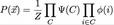## July 16, 2004

### On the Equivalence of Directed and Undirected Graphical ModelsA directed graphical model can be converted to an undirected graphical model through a process called moralization. Graphically, moralization is accomplished by creating an undirected clique for every node in the directed graph and it's parents.

A directed graph has a conditional probability table (CPT) for every child given its parents. In the undirected graph this CPT becomes the clique potential function, psi. Each node in the undirected graph also has a node potential. If the node had a prior in the directed graph, that becomes the node potential in the undirected graph. Otherwise it is uniformly a constant.

The joint probability function in the directed case goes from:To the undirected case:Which translates to: the joint probability of a setting of variables, x, is equal to the product of the clique potential functions evaluated with the relevant elements of x passed as parameters, times the node potential functions of all the individual nodes given their queried setting. This has to be scaled by a normalization factor because the original conditional probability tables, while normalized for a given setting of the parent nodes, are not normalized across the joint distribution of parents and child.

Finally it is worth noting that the moralized graph can represent a larger range of probability distributions than the original directed graph. This might be relevant if you desired to learn the parameters of a directed graph. If you did this in a particular way, namely by moralizing the graph and then learning parameters on that graph, the resulting learned graph might not be representable in the original directed graph.

Posted by djp3 at July 16, 2004 1:14 PM | TrackBack (0)
Comments
Post a comment